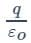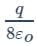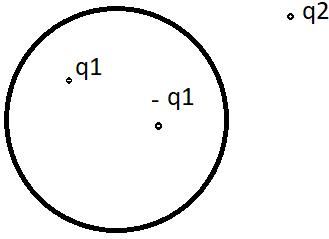JEE  >  Test - Electric Flux and gauss's law

# Test - Electric Flux and gauss's law

Test Description

## 10 Questions MCQ Test Physics For JEE | Test - Electric Flux and gauss's law

Test - Electric Flux and gauss's law for JEE 2023 is part of Physics For JEE preparation. The Test - Electric Flux and gauss's law questions and answers have been prepared according to the JEE exam syllabus.The Test - Electric Flux and gauss's law MCQs are made for JEE 2023 Exam. Find important definitions, questions, notes, meanings, examples, exercises, MCQs and online tests for Test - Electric Flux and gauss's law below.
Solutions of Test - Electric Flux and gauss's law questions in English are available as part of our Physics For JEE for JEE & Test - Electric Flux and gauss's law solutions in Hindi for Physics For JEE course. Download more important topics, notes, lectures and mock test series for JEE Exam by signing up for free. Attempt Test - Electric Flux and gauss's law | 10 questions in 10 minutes | Mock test for JEE preparation | Free important questions MCQ to study Physics For JEE for JEE Exam | Download free PDF with solutions
 1 Crore+ students have signed up on EduRev. Have you?
Test - Electric Flux and gauss's law - Question 1

### What is the dimension of electric flux?

Detailed Solution for Test - Electric Flux and gauss's law - Question 1

Electric flux = electric field intensity x area. The dimension of field intensity is [M L T-3 I-1] and the dimension of the area is [L2]. Therefore, the dimension of flux = [M L3 T-3 I-1]. This can also be justified as flux=potential x length. By putting the dimensions of potential and length, we can get the same result.

Test - Electric Flux and gauss's law - Question 2

### An electric charge q is placed at one of the corner points of a cube of side a. What will be the net outwards flux coming out of the cube due to the point charge?

Detailed Solution for Test - Electric Flux and gauss's law - Question 2

We can assume 8 imaginary cubes, all of side a placed together, sharing a common corner point and forming a bigger cube of side 2a. The point charge is placed at the common point of the cubes. According to Gauss’s Law, net electric flux coming out of the bigger cube =Therefore, flux coming out from each of the smaller cubes i.e., cubes of side a is.

Test - Electric Flux and gauss's law - Question 3

### A non-conducting sphere has uniform charge density in it. The electric field at a point inside the sphere will be _______ Zero

Detailed Solution for Test - Electric Flux and gauss's law - Question 3

In the case of a non-conducting sphere, an electric charge is uniformly distributed throughout the sphere. Inside the sphere, the electric field at a point will be only due to the electric charge inside that point. There will be no effect of the remaining charge outside that point. In the case of the conducting sphere, the electric field at every point inside the sphere is zero.

Test - Electric Flux and gauss's law - Question 4

What is the number of electric field lines coming out from a 1C charge?

Detailed Solution for Test - Electric Flux and gauss's law - Question 4

According to Gauss’s Law, the total number of electric field lines coming out of a charge q is =where εo is the absolute permittivity of air. Its value is 8.85 * 10-12. Therefore the number of lines coming out from a 1C charge = 1/8.85 * 10-12.

Test - Electric Flux and gauss's law - Question 5

Gauss Law can be used to compute which of the following?

Detailed Solution for Test - Electric Flux and gauss's law - Question 5

Gauss law relates the electric flux density and the charge density. Thus it can be used to compute the radius of the Gaussian surface. Permittivity and permeability are constants for a particular material.

Test - Electric Flux and gauss's law - Question 6

Electric field outside this Gaussian surface will be __________Detailed Solution for Test - Electric Flux and gauss's law - Question 6

According to Gauss’s Law, the electric field outside a closed surface will be only because of the total charge enclosed inside the surface. In this case, there are two positive and negative charges of the same magnitude inside the surface. Therefore the net charge inside the surface is zero. Therefore, the electric field outside the surface will also be zero. There will be no effect of the second charge q2

Test - Electric Flux and gauss's law - Question 7

Electric flux will be maximum if the angle between the field lines and area vector is ______

Detailed Solution for Test - Electric Flux and gauss's law - Question 7

We know that the electric field and area both are vector quantity and the electric flux is expressed as E.s (taking dot product). But if the angle between the two vectors is θ then the formula becomes E-.s-.cosθ. Cosθ will be maximum if theta is zero, in all other cases, the value of Cosθ is less than 1. Therefore flux will be the maximum if the angle is 0 degrees.

Test - Electric Flux and gauss's law - Question 8

If a charge is placed outside a closed surface, flux due to that charge inside the surface will be ________

Detailed Solution for Test - Electric Flux and gauss's law - Question 8

According to Gauss’s principle, if there is no charge bound inside a surface, net electric flux coming out of the surface will always be 0. In this case, the charge is kept outside the surface, so it will generate no field lines and hence no flux inside the surface. The situation will be the same if an electric dipole is placed inside a surface as the dipole has equal positive and negative charges; the total charge inside the surface becomes 0.

Test - Electric Flux and gauss's law - Question 9

Gauss law can be evaluated in which coordinate system?

Detailed Solution for Test - Electric Flux and gauss's law - Question 9

The Gauss law exists for all materials. Depending on the Gaussian surface of the material, we take the coordinate system accordingly. Suppose if the material is a coaxial cable, the Gaussian surface is in the form of a cylinder. Thus we take the cylinder/circular coordinate system.

Test - Electric Flux and gauss's law - Question 10

Three charged cylindrical sheets are present in three spaces with σ = 5 at R = 2m, σ = -2 at R = 4m and σ = -3 at R = 5m. Find the flux density at R = 1m.

Detailed Solution for Test - Electric Flux and gauss's law - Question 10

Since 1m does not enclose any cylinder (three Gaussian surfaces of radius 2m, 4m, 5m exists), the charge density and charge becomes zero according to Gauss law. Thus flux density is also zero.

## Physics For JEE

258 videos|633 docs|256 tests
 Use Code STAYHOME200 and get INR 200 additional OFF Use Coupon Code
Information about Test - Electric Flux and gauss's law Page
In this test you can find the Exam questions for Test - Electric Flux and gauss's law solved & explained in the simplest way possible. Besides giving Questions and answers for Test - Electric Flux and gauss's law, EduRev gives you an ample number of Online tests for practice

## Physics For JEE

258 videos|633 docs|256 tests